The Arduino C Programming Language Basics

1. Start the Arduino IDE

Go to the start menu, type 'arduino', click the App shows up as follows: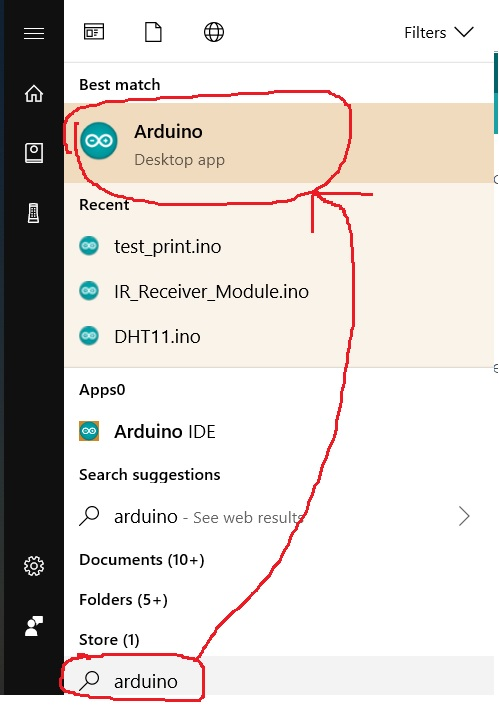Here is the next window you will see on your side: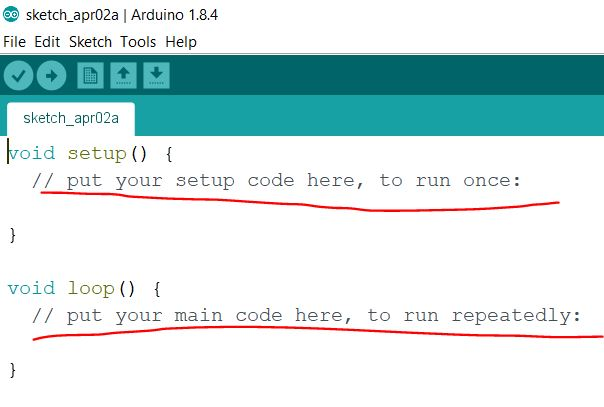The Arduino IDE already put two functions there for you to fill up with your code. One is 'void setup()', the other is 'void loop()'. They both have a title of 'void' because they are not returning anything after the function executed. Well, it's fine to not understand what is 'return' now.

The 'setup()' function will run for only once but the 'loop()' function will be ran repeatedly. This enables the automation of an electronics application. A lot of times, if you want to automate a procedure, you must re-check the status and provide a corresponding response. This is how computers automate things. It is a little different from human's brain.

2. The Arduino C language basics

2.1 Variables and Data Types

In arudino programming in C language

In your sketches, most of the times you want to store data and perform some type of calculation. Counting the number of times a button is pushed, storing the voltage on an analog pin, or performing a complex mathematical calculation with vectors: require data to be calculated and stored. This data is saved in a variable, a memory location that can be changed as required. By declaring a variable, you are asking the compiler to allocate a specific amount of memory, depending on the data typeData types and variables are used to store the information. When we declare variable we are actually telling compiler to allocate a specific amount of memory, depending on data type.

Below is a list of the data types commonly seen in Arduino, with the memory size of each in parentheses after the type name. Note: signed variables allow both positive and negative numbers, while unsigned variables allow only positive values.

* boolean (8 bit) - simple logical true/false
* byte (8 bit) - unsigned number from 0-255
* char (8 bit) - signed number from -128 to 127. The compiler will attempt to interpret this data type as a character in some circumstances, which may yield unexpected results
* unsigned char (8 bit) - same as 'byte'; if this is what you're after, you should use 'byte' instead, for reasons of clarity
* word (16 bit) - unsigned number from 0-65535
* unsigned int (16 bit)- the same as 'word'. Use 'word' instead for clarity and brevity
* int (16 bit) - signed number from -32768 to 32767. This is most commonly what you see used for general purpose variables in Arduino example code provided with the IDE
* unsigned long (32 bit) - unsigned number from 0-4,294,967,295. The most common usage of this is to store the result of the millis() function, which returns the number of milliseconds the current code has been running
* long (32 bit) - signed number from -2,147,483,648 to 2,147,483,647
* float (32 bit) - signed number from -3.4028235E38 to 3.4028235E38. Floating point on the Arduino is not native; the compiler has to jump through hoops to make it work. If you can avoid it, you should. We'll touch on this later.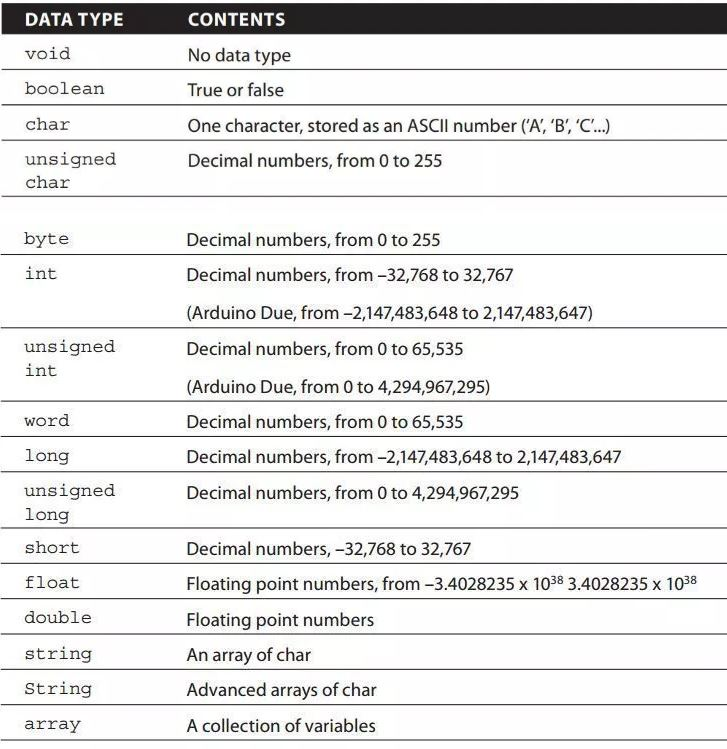2.2 Operators

(More details can be found on Arduino's website:
https://www.arduino.cc/reference/en/)

##### Arithmetic Operators
% (remainder)
* (multiplication)
- (subtraction)
/ (division)
= (assignment operator)
##### Comparison Operators
!= (not equal to)
< (less than)
<= (less than or equal to)
== (equal to)
> (greater than)
>= (greater than or equal to)
##### Boolean Operators
! (logical not)
&& (logical and)
|| (logical or)
##### Pointer Access Operators
& (reference operator)
* (dereference operator)
##### Bitwise Operators
& (bitwise and)
<< (bitshift left)
>> (bitshift right)
^ (bitwise xor)
| (bitwise or)
~ (bitwise not)
##### Compound Operators
%= (compound remainder)
&= (compound bitwise and)
*= (compound multiplication)
++ (increment)
-- (decrement)
-= (compound subtraction)
/= (compound division)
^= (compound bitwise xor)
|= (compound bitwise or)
Sketch
break
continue
do...while
else
for
goto
if
return
switch...case
while
##### Further Syntax
#define (define)
#include (include)
/* */ (block comment)
// (single line comment)
; (semicolon)
{} (curly braces)

2.3 Control Structures

Control Structure in Arduino programming in C Language

if Statement in C Language:
The if statement is used to detect if an expression is equal to a result
Following versions are used.
One is:

if (expression)
{
statement;
}

Two conditions are as follows:

if (expression)
{
do_this;
}
else
{
do_that;
}

We can mix several if else statements using:

if (expression)
{
do_this;
}
else if (expression)
{
do_that;
}

Try to evaluate a given program:

int var1 = 42;
if (var1 == 42)
{
run_this; // var1 equals 42; this function will be executed
}
else
{
run_that; //This one will not
}
if  (var1 < 50)
{
run_another_function; //This will be run, since 42 is less than 50
}

Switch Statement in Arduino programming in C Language

When we need to check a large number of conditions and need to execute a statement according to a specific condition, we use switch/case statement. It is like if statement. A switch statement the value of variable, and execute a different case statement depending on value.

switch(button)

{

case 1:

turn_on_lights();

break;

case 2:

if (blinds_up == false)

{

raise_blinds();

blinds_up = true;

}

break;

case 3:

----

}

Break: at the end of each instruction actually tells compiler to stop the execution. If 'break;' is not used, it will continue executing the case instruction. For example if button 4, 5, 6 do same task you can write

The 'While Loop' in Arduino programming

It is one of the basic loops of C language; it will execute same code again and again until a condition is specified. As long as condition is true it will continue running same code and keep checking the condition at the end of each loop.

while (button == false)
{
button = check_status(pin4);
}

In this example, the function check status runs until it returns true. When that happens, the variable button becomes true, and the while loop will be broken. It might be within a few milliseconds, or the system might wait indefinitely.

The 'For Loop' in Arduino programming

In cases in which you need a portion of code to loop an exact number of times, the for loop is used. It is similar to while, only it is written differently. The for loop keeps track of the number of times it has run.

for (expression1; expression2; expression3)

{

instructions;

instructions;

}

It requires three expressions:

• expression1 is the initializer; it will initialize a variable.
• expression2 is the conditional expression; as long as this condition is true, the loop keeps on executing.
• expression3 is the modifier; when a loop is completed, this action is performed.

For example:

for (int i = 0; i < 10; i++)

{

myfunc(i);

}

In this example, a variable is defined with the name i. The variable is set to zero, and each time the function myfunc is run, i is increased by one. Finally, when i reaches 10, the loop stops before running myfunc. This saves you from writing out all the commands one by one like this:
myfunc(0);
myfunc(1);

myfunc(8);
myfunc(9);

Bit masks are used to access specific bits in a byte of data. This is often useful as a method of iteration, for example when sending a byte of data serially out a single pin. In this example the pin needs to change it's state from high to low for each bit in the byte to be transmitted. This is accomplished using what are known as bitwise operations and a bit mask. Bitwise operations perform logical functions that take affect on the bit level. Standard bitwise operations include AND (&) OR (|) Left Shift (<<) and Right Shift (>>).

The AND (&) operator will result in a 1 at each bit position where both input values were 1. For example: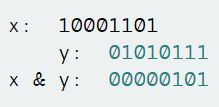The OR (|) operator (also known as Inclusive Or) will result in a 1 at each bit position where either input values were 1. For example: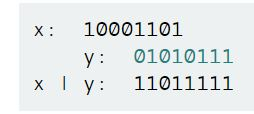The Left Shift (<<) operator will shift a value to the left the specified number of times. For example: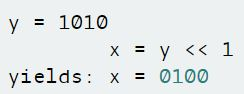All the bits in the byte get shifted one position to the left and the bit on the left end drops off.

The Right Shift (>>) operator works identically to left shift except that it shifts the value to the right the specified number of times For example:All the bits in the byte get shifted one position to the right and the bit on the right end drops off.

3. Run your code on the Arduino board

Since all the programs are supposed to be ran on the Arduino board so we need to download the code to Arduino, run it using Arduino's CPU and return the result to our PC to see the results.
Keep in mind that the Arduino board has a Atmel Mega 328 P microcontroller unit (MCU), which has a CPU in side. You also have a CPU in your PC but it is way more complicated than the CPU in the MCU chip.

We are using the USB cable to talk to the MCU thorugh our PC. We can easily download the Arduino C code to the board and let it run there. However, if we want to see the result after the computation in the MCU, we need the results to be sent back to the PC. Therefore we need the USB cable to connect the board and the PC.

The communication between the board and the PC is called UART or 'Serial Communication'. It is fine to not understand what 'Serial' stands for. Just need to know that you need the USB cable to send signals to and receive signals from the board.

You need the following steps before you can talk to the board using 'Serial Communication'.

Go to 'Tools' in the menu bar, make sure you selected the 'Arduino/Genuino Uno' Port. I have COM34 for me, your COM port number will be different from mine, just select it.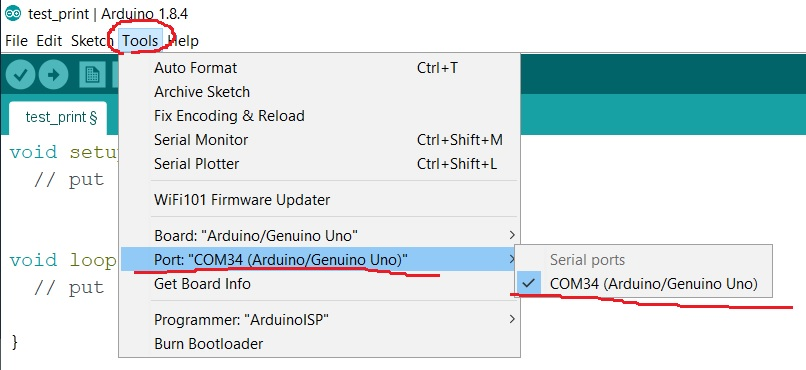Connect the board and the PC using the USB cable.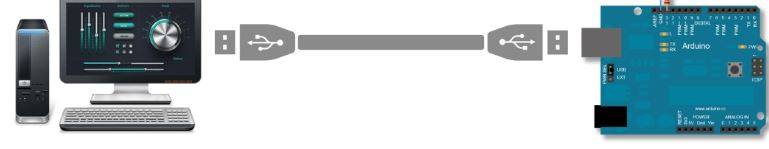Open your Arduino IDE and start a new file: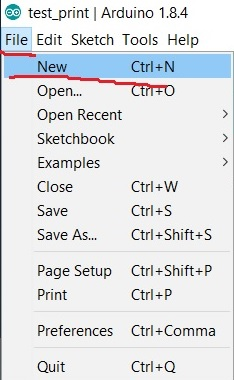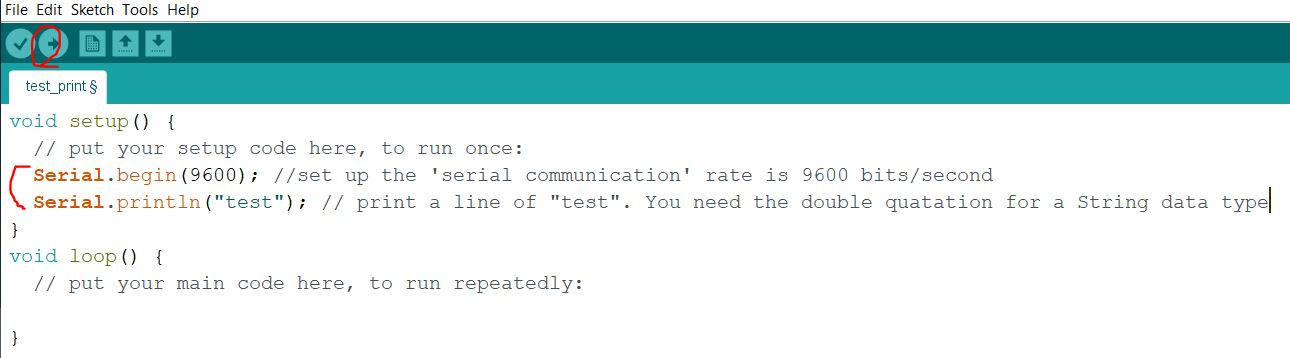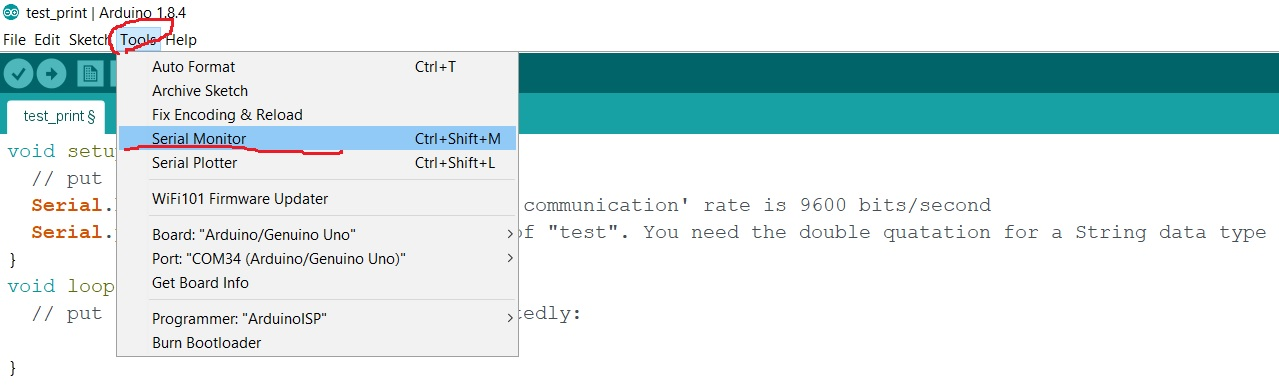You'll see that in the serial monitor, the board will send 'test' to you as you defined in the code.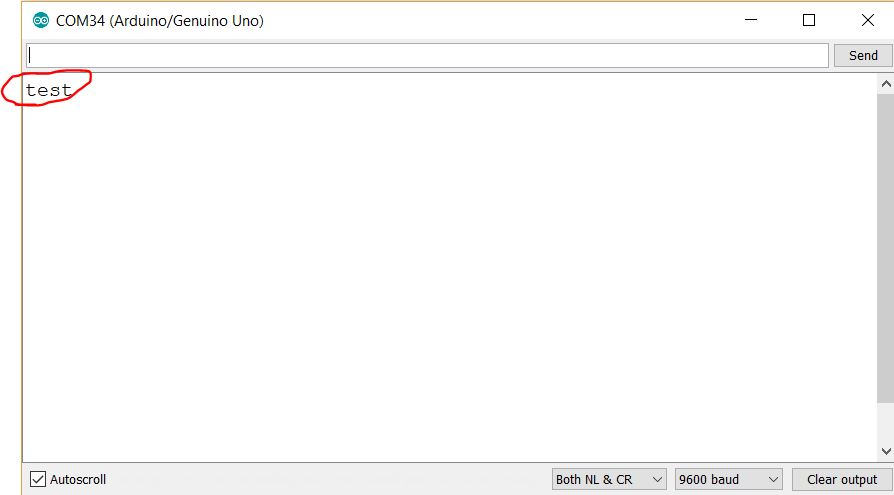Since the 'setup()' function will be executed for only once, so you only have one 'test' being printed out.

If you change the code as follows: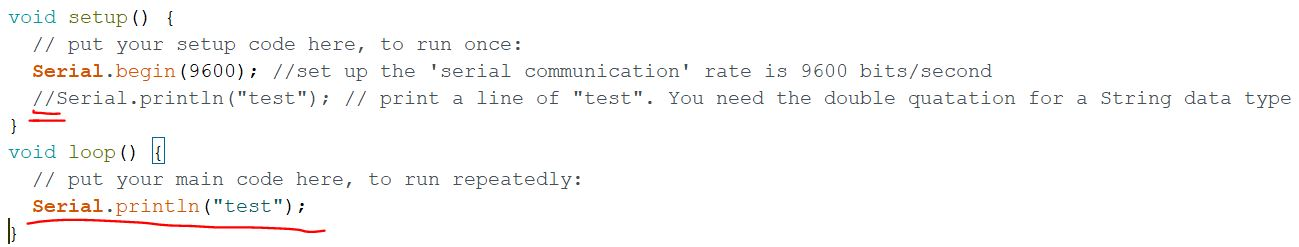You'll see the results as follows: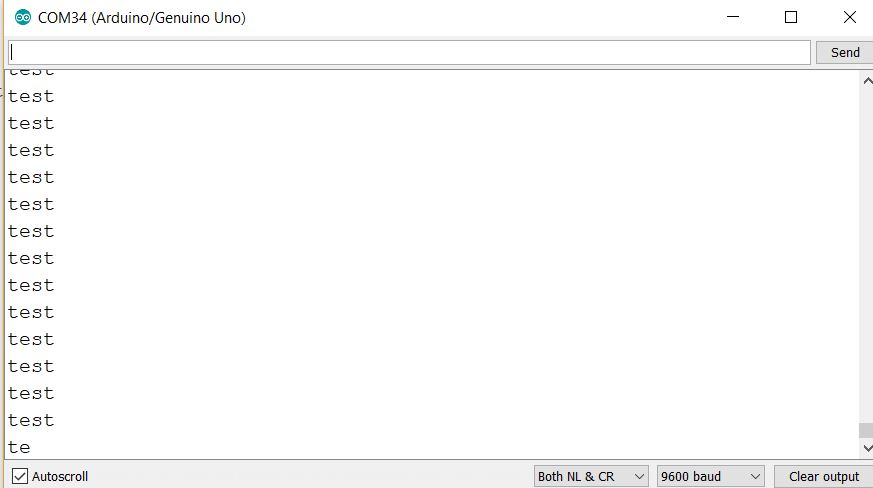However, this is a dead loop. If you don't unplug the board, it will being executed permanently.

You can use the following code to bring it to the end after the first execution:Even though it is a dead loop, but I'll terminate the serial communication in in the first loop. You will only get 1 "test" in the monitor by using this code.

4. Test the code using Arduino and the serial monitor from your PC.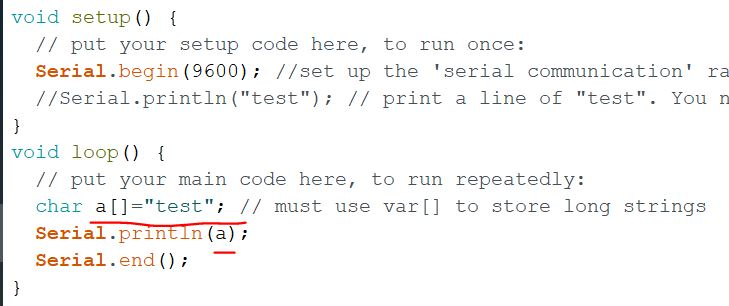You'll get the expected result: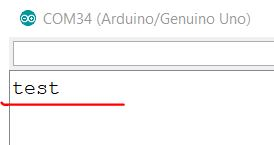And from the results below, we know that we do have CPU in the Arduino chip. It is able to perform these arithmetic operations:One more example: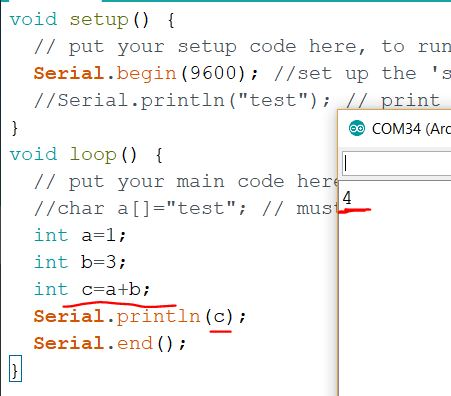Let's test some logic operations: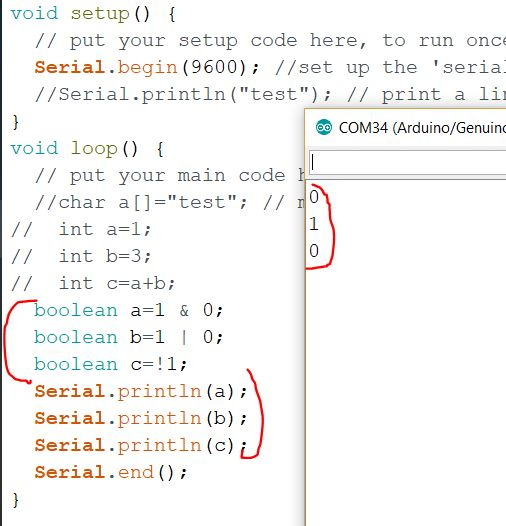I changed one of the variables into an 'int' type and it didn't affect the results:5. Control structures and loops

Use the 'if' statement to make a judgement before the execution of the code.

Syntax:

if (expression)
{
do_this;
}
else if (expression)
{
do_that;
}
else (expression)
{
do_that;
}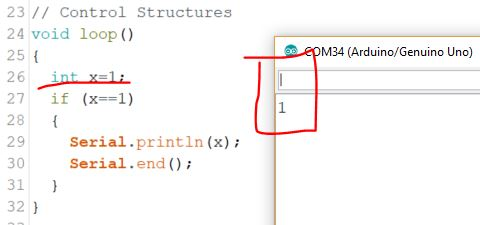The 'For' loops:

Syntax:

for (expression1; expression2; expression3)

{

instructions;

instructions;

}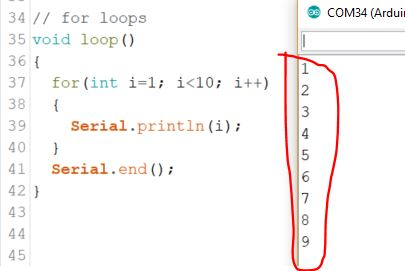Think about that: where should you modify if you want to print all the numbers from 1 - 10?

An example have both the 'if' statement and the 'for' loop: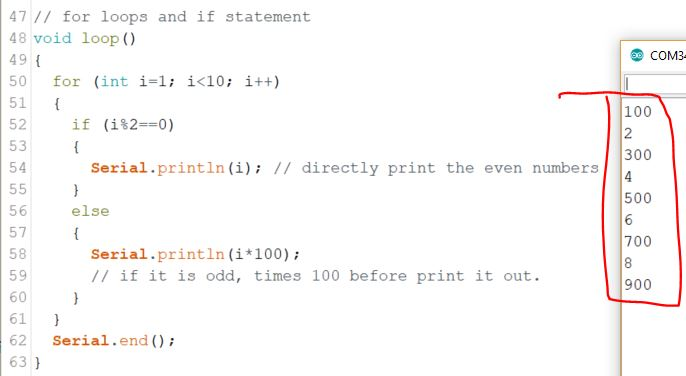The 'while' loops:

Syntax:

while (button == false)
{
button = check_status(pin4);
}

The 'while' loop can do the looping job as well but the format is a little different.
You must be wondering when to use a 'for' loop and when to use a 'while' loop:You have a 'void loop()' function already, why do you still need a 'while' loop to do the job?

The 'void loop()' function does not have a conditional judgement to control the execution. You need a condition to check to control the loop.

So the question is will the 'void loop()' function run forever?

The anwser is 'YES', as long as you get your board powered up, the 'void loop()' function will start running to check if there is any changes/updates in the condition. If there are any, the system will run the corresponding code for this changed condition.

However, here, after the coutner 'i' reaches 10 for the first loop in the 'void loop()' function, the next time it enters into this 'void loop()' function, 'i' doesn't satisfy the condition any more for the 'while' loop, so the program inside the 'while' loop won't run.

The 'switch' statement:

Syntax:

switch (button)

{

case 4:

case 6:

case 8:

//code to be run

break;

}

Look at the following example. Why the 'Now it is 6' will be executed for twice?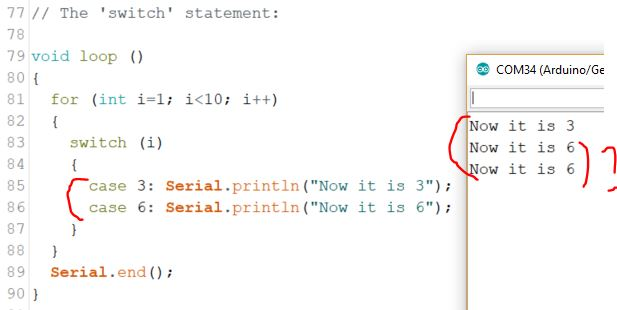The reason is the 'switch' statement works in the following way: the 'switch (i)' line detects the value of 'i' and enters the specific case directly and then run all the rest of the 'cases' below this entry. So, it entered 'case 3' and then run the 'case 6' after this. In the next a few 'for' loops, when 'i' hits '6', it will directly enter the 'switch' statement at 'case 6' and run it again. Therefore, you see two of 'Now it is 6'.

In order to avoid this situation, we need to use the 'break' statement to exit the 'switch' statement after every 'case' so the code below that entry will not be executed.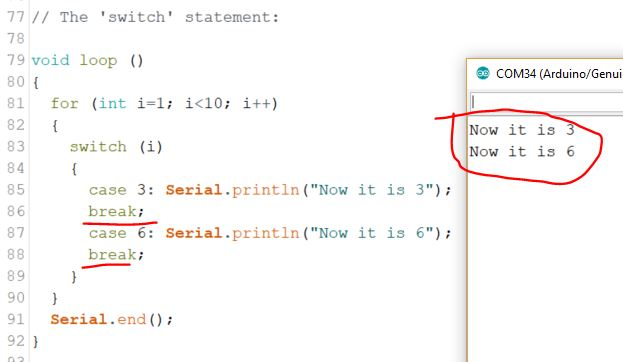1. Repeat all the examples in Sections 3, 4, and 5. Make snapshots, save them as figures for the report. Add captions for the figrues to explain the results. The example caption for a figure: "Figure 1: The 'for' loop example". Add some narative contents in the report to briefly explain the results.

2. There is another control function not mentioned in this tutorial - 'do while'. The Syntax is:
do {
something;
} while (condition);

Create one or a few examples to demonstrate how this function works. Include this in your report. (include figures/captions and descriptions for this design).

3. Use HEX numbers to represent multiple binary bits is very conventient. The format is '0xFFF', which starts with '0x' (zero ex) and followed by the HEX number.
I have the following code executed in Arduino. Repeat it on your side and explain why the result is 4096?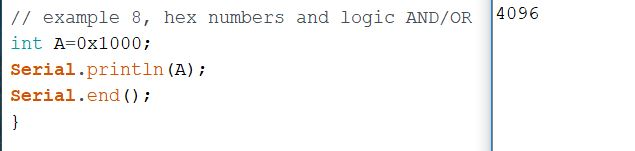4. Use the HEX format (0x.....) (16 bits) to represent the following numbers: (keep in mind there must be 16 bits in your result)
(a) 1000 1111 0011 0101 (binary)
(b) 55 (decimal)
(c) 11 (binary)

5. Convert the following HEX format into Binary:
(a) 0xFFFF
(b) 0x3210
(c) 0xABCD

6. The following example can transmit every single bit in a number to ONE specific pin on the Arduino board. Use the similar mathod (bit masks) to display a 5-bit binary number on FIVE different LEDs (in parallel).
You must use the a mask to fetch every single digit in the data number. After every digit is rechieved, store it in a location of an Array data type (tutorial here:
https://www.arduino.cc/reference/en/language/variables/data-types/array/). When the rechieving is done, display all the digits stored in an array to 5 LEDs in parallel (at the same time). (use the LEDs to display every single bit in a data).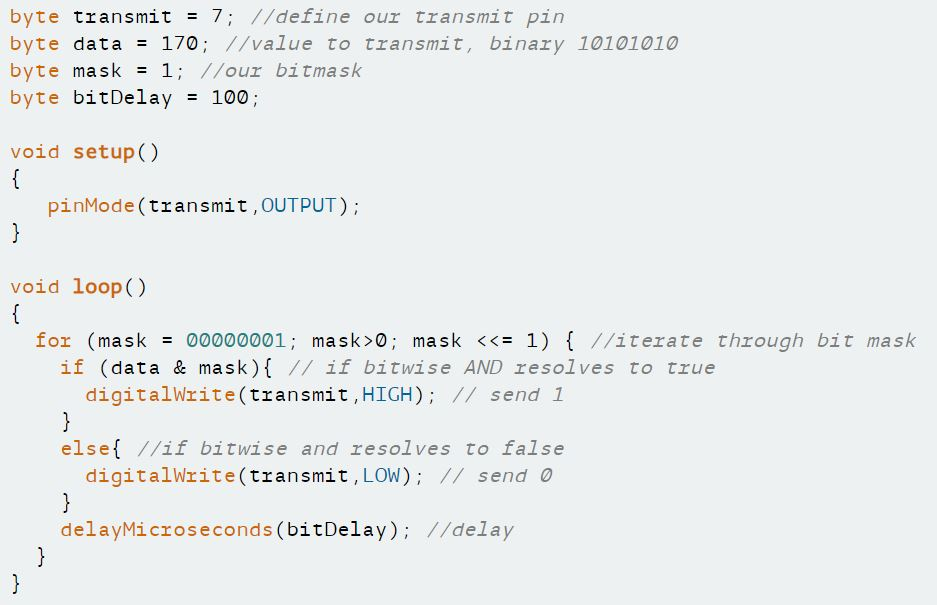-------------------------Resistor Code (I'll quiz you on this)--------------------------------